Anúncio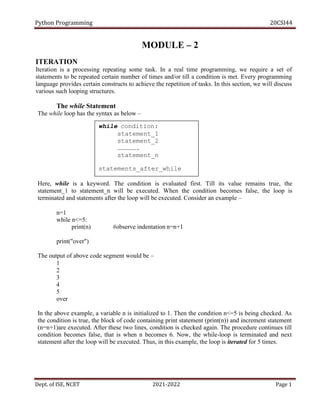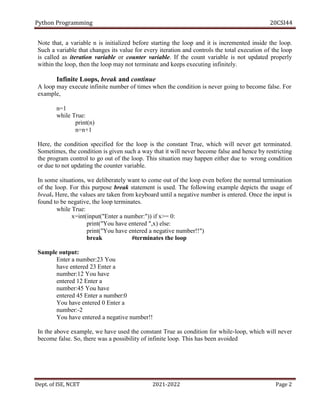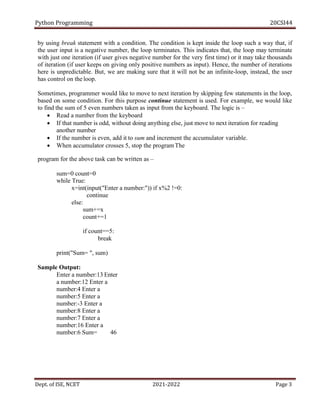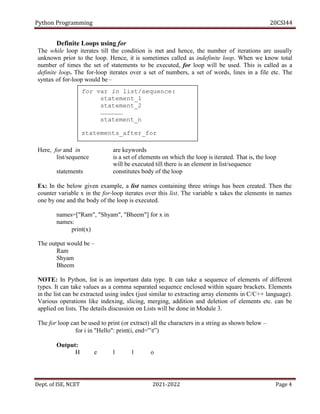Anúncio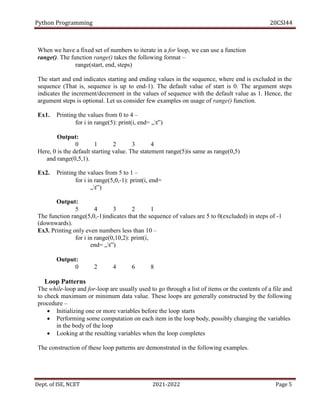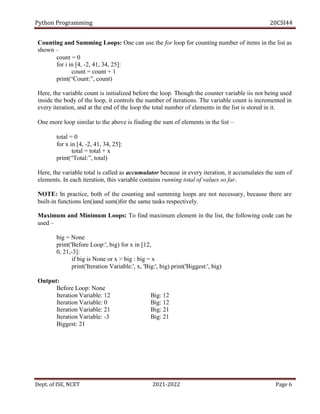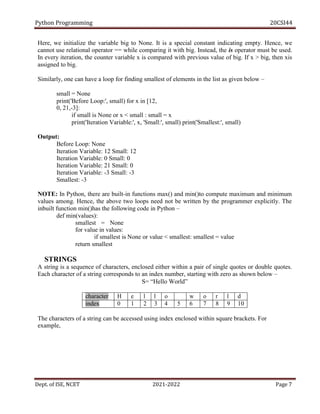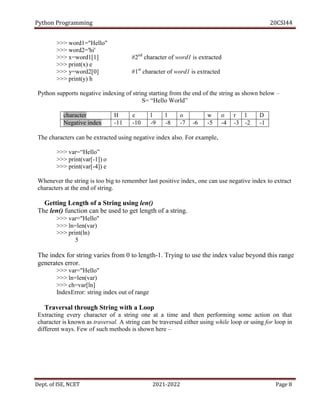Anúncio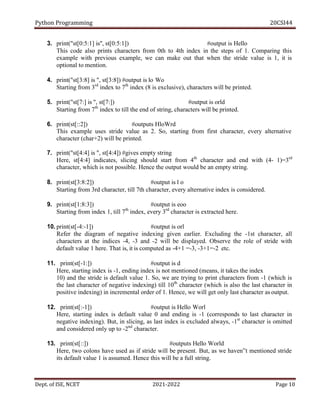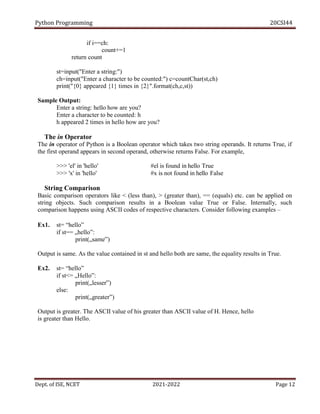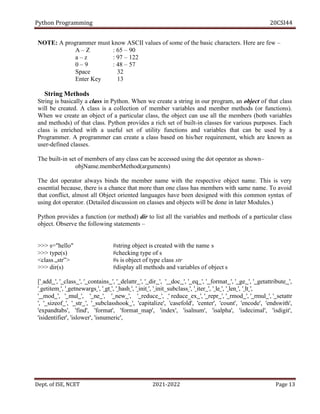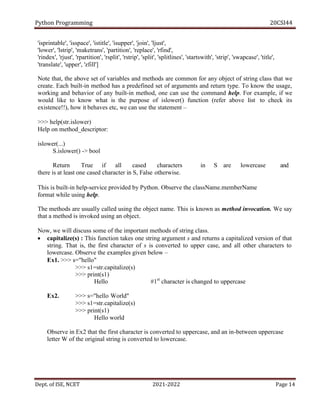Anúncio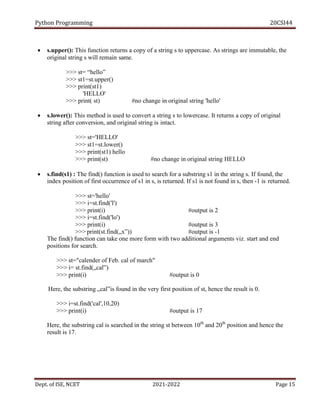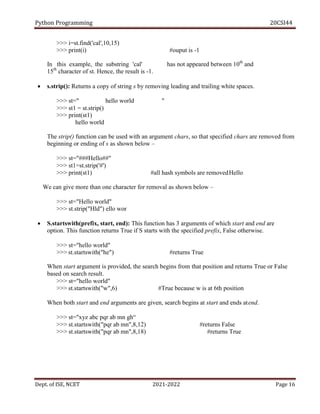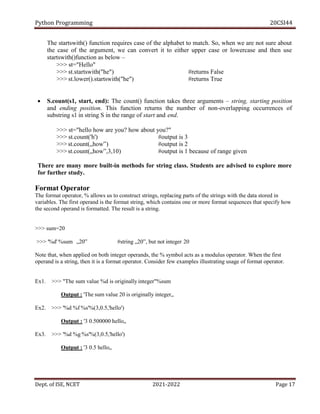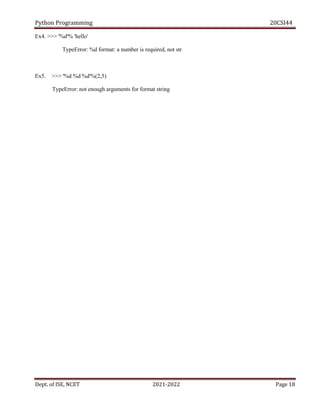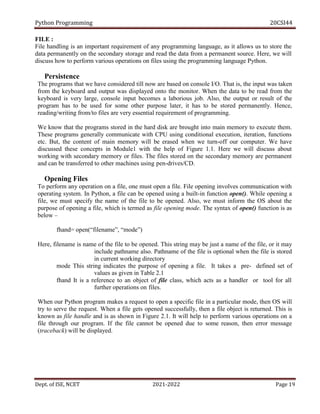Anúncio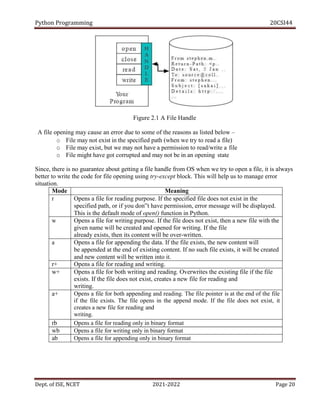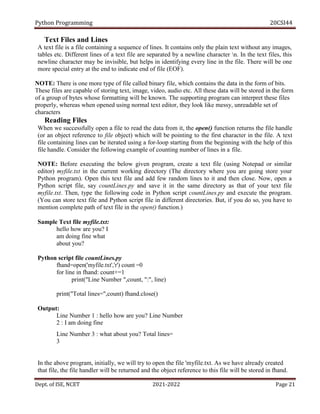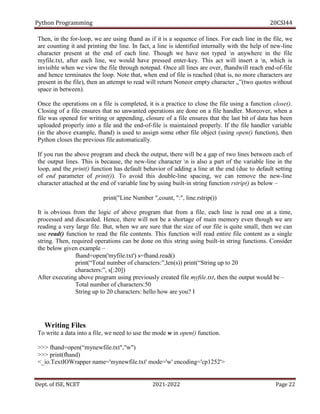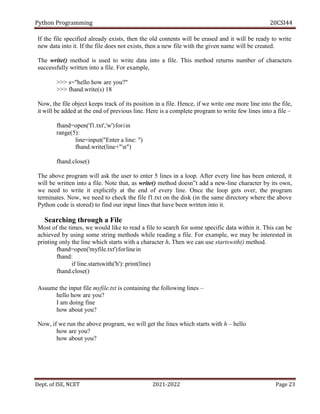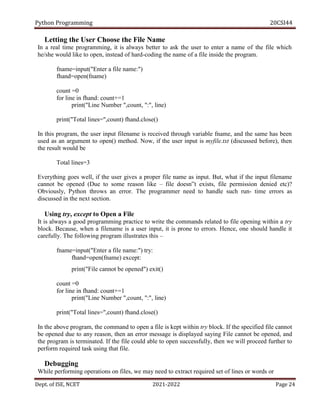Anúncio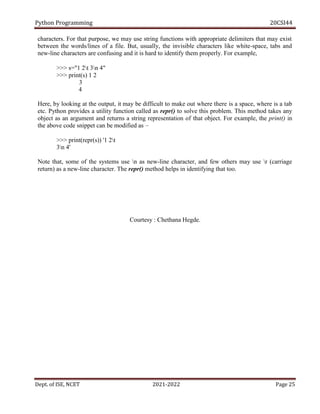Próximos SlideShares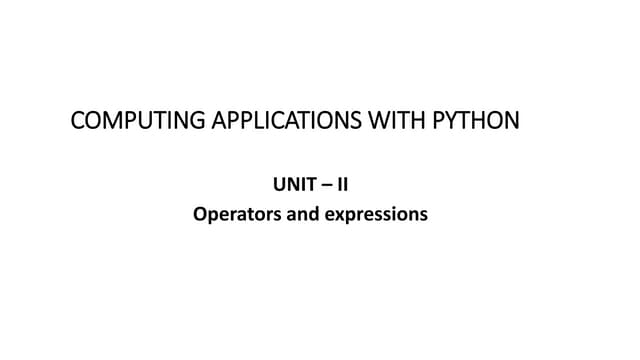Unit - 2 CAP.pptx
Carregando em ... 3
1 de 25
Anúncio

### Python_Module_2.pdf

1. Python Programming 20CSI44 Dept. of ISE, NCET 2021-2022 Page 1 while condition: statement_1 statement_2 ……………. statement_n statements_after_while ITERATION MODULE – 2 Iteration is a processing repeating some task. In a real time programming, we require a set of statements to be repeated certain number of times and/or till a condition is met. Every programming language provides certain constructs to achieve the repetition of tasks. In this section, we will discuss various such looping structures. The while Statement The while loop has the syntax as below – Here, while is a keyword. The condition is evaluated first. Till its value remains true, the statement_1 to statement_n will be executed. When the condition becomes false, the loop is terminated and statements after the loop will be executed. Consider an example – n=1 while n<=5: print(n) #observe indentation n=n+1 print("over") The output of above code segment would be – 1 2 3 4 5 over In the above example, a variable n is initialized to 1. Then the condition n<=5 is being checked. As the condition is true, the block of code containing print statement (print(n)) and increment statement (n=n+1)are executed. After these two lines, condition is checked again. The procedure continues till condition becomes false, that is when n becomes 6. Now, the while-loop is terminated and next statement after the loop will be executed. Thus, in this example, the loop is iterated for 5 times.
2. Python Programming 20CSI44 Dept. of ISE, NCET 2021-2022 Page 2 Note that, a variable n is initialized before starting the loop and it is incremented inside the loop. Such a variable that changes its value for every iteration and controls the total execution of the loop is called as iteration variable or counter variable. If the count variable is not updated properly within the loop, then the loop may not terminate and keeps executing infinitely. Infinite Loops, break and continue A loop may execute infinite number of times when the condition is never going to become false. For example, n=1 while True: print(n) n=n+1 Here, the condition specified for the loop is the constant True, which will never get terminated. Sometimes, the condition is given such a way that it will never become false and hence by restricting the program control to go out of the loop. This situation may happen either due to wrong condition or due to not updating the counter variable. In some situations, we deliberately want to come out of the loop even before the normal termination of the loop. For this purpose break statement is used. The following example depicts the usage of break. Here, the values are taken from keyboard until a negative number is entered. Once the input is found to be negative, the loop terminates. while True: x=int(input("Enter a number:")) if x>= 0: print("You have entered ",x) else: print("You have entered a negative number!!") break #terminates the loop Sample output: Enter a number:23 You have entered 23 Enter a number:12 You have entered 12 Enter a number:45 You have entered 45 Enter a number:0 You have entered 0 Enter a number:-2 You have entered a negative number!! In the above example, we have used the constant True as condition for while-loop, which will never become false. So, there was a possibility of infinite loop. This has been avoided
3. Python Programming 20CSI44 Dept. of ISE, NCET 2021-2022 Page 3 by using break statement with a condition. The condition is kept inside the loop such a way that, if the user input is a negative number, the loop terminates. This indicates that, the loop may terminate with just one iteration (if user gives negative number for the very first time) or it may take thousands of iteration (if user keeps on giving only positive numbers as input). Hence, the number of iterations here is unpredictable. But, we are making sure that it will not be an infinite-loop, instead, the user has control on the loop. Sometimes, programmer would like to move to next iteration by skipping few statements in the loop, based on some condition. For this purpose continue statement is used. For example, we would like to find the sum of 5 even numbers taken as input from the keyboard. The logic is –  Read a number from the keyboard  If that number is odd, without doing anything else, just move to next iteration for reading another number  If the number is even, add it to sum and increment the accumulator variable.  When accumulator crosses 5, stop the programThe program for the above task can be written as – sum=0 count=0 while True: x=int(input("Enter a number:")) if x%2 !=0: continue else: sum+=x count+=1 if count==5: break print("Sum= ", sum) Sample Output: Enter a number:13 Enter a number:12 Enter a number:4 Enter a number:5 Enter a number:-3 Enter a number:8 Enter a number:7 Enter a number:16 Enter a number:6 Sum= 46
4. Python Programming 20CSI44 Dept. of ISE, NCET 2021-2022 Page 4 for var in list/sequence: statement_1 statement_2 ……………… statement_n statements_after_for Definite Loops using for The while loop iterates till the condition is met and hence, the number of iterations are usually unknown prior to the loop. Hence, it is sometimes called as indefinite loop. When we know total number of times the set of statements to be executed, for loop will be used. This is called as a definite loop. The for-loop iterates over a set of numbers, a set of words, lines in a file etc. The syntax of for-loop would be– Here, for and in are keywords list/sequence is a set of elements on which the loop is iterated. That is, the loop will be executed till there is an element in list/sequence statements constitutes body of the loop Ex: In the below given example, a list names containing three strings has been created. Then the counter variable x in the for-loop iterates over this list. The variable x takes the elements in names one by one and the body of the loop is executed. names=["Ram", "Shyam", "Bheem"] for x in names: print(x) The output would be – Ram Shyam Bheem NOTE: In Python, list is an important data type. It can take a sequence of elements of different types. It can take values as a comma separated sequence enclosed within square brackets. Elements in the list can be extracted using index (just similar to extracting array elements in C/C++ language). Various operations like indexing, slicing, merging, addition and deletion of elements etc. can be applied on lists. The details discussion on Lists will be done in Module 3. The for loop can be used to print (or extract) all the characters in a string as shown below – for i in "Hello": print(i, end=‟t‟) Output: H e l l o
5. Python Programming 20CSI44 Dept. of ISE, NCET 2021-2022 Page 5 When we have a fixed set of numbers to iterate in a for loop, we can use a function range(). The function range() takes the following format – range(start, end, steps) The start and end indicates starting and ending values in the sequence, where end is excluded in the sequence (That is, sequence is up to end-1). The default value of start is 0. The argument steps indicates the increment/decrement in the values of sequence with the default value as 1. Hence, the argument steps is optional. Let us consider few examples on usage of range() function. Ex1. Printing the values from 0 to 4 – for i in range(5): print(i, end= „t‟) Output: 0 1 2 3 4 Here, 0 is the default starting value. The statement range(5)is same as range(0,5) and range(0,5,1). Ex2. Printing the values from 5 to 1 – for i in range(5,0,-1): print(i, end= „t‟) Output: 5 4 3 2 1 The function range(5,0,-1)indicates that the sequence of values are 5 to 0(excluded) in steps of -1 (downwards). Ex3. Printing only even numbers less than 10 – for i in range(0,10,2): print(i, end= „t‟) Output: 0 2 4 6 8 Loop Patterns The while-loop and for-loop are usually used to go through a list of items or the contents of a file and to check maximum or minimum data value. These loops are generally constructed by the following procedure –  Initializing one or more variables before the loop starts  Performing some computation on each item in the loop body, possibly changing the variables in the body of the loop  Looking at the resulting variables when the loop completes The construction of these loop patterns are demonstrated in the following examples.
6. Python Programming 20CSI44 Dept. of ISE, NCET 2021-2022 Page 6 Counting and Summing Loops: One can use the for loop for counting number of items in the list as shown – count = 0 for i in [4, -2, 41, 34, 25]: count = count + 1 print(“Count:”, count) Here, the variable count is initialized before the loop. Though the counter variable iis not being used inside the body of the loop, it controls the number of iterations. The variable count is incremented in every iteration, and at the end of the loop the total number of elements in the list is stored in it. One more loop similar to the above is finding the sum of elements in the list – total = 0 for x in [4, -2, 41, 34, 25]: total = total + x print(“Total:”, total) Here, the variable total is called as accumulator because in every iteration, it accumulates the sum of elements. In each iteration, this variable contains running total of values so far. NOTE: In practice, both of the counting and summing loops are not necessary, because there are built-in functions len()and sum()for the same tasks respectively. Maximum and Minimum Loops: To find maximum element in the list, the following code can be used – big = None print('Before Loop:', big) for x in [12, 0, 21,-3]: if big is None or x > big : big = x print('Iteration Variable:', x, 'Big:', big) print('Biggest:', big) Output: Before Loop: None Iteration Variable: 12 Big: 12 Iteration Variable: 0 Big: 12 Iteration Variable: 21 Big: 21 Iteration Variable: -3 Big: 21 Biggest: 21
7. Python Programming 20CSI44 Dept. of ISE, NCET 2021-2022 Page 7 Here, we initialize the variable big to None. It is a special constant indicating empty. Hence, we cannot use relational operator == while comparing it with big. Instead, the is operator must be used. In every iteration, the counter variable x is compared with previous value of big. If x > big, then xis assigned to big. Similarly, one can have a loop for finding smallest of elements in the list as given below – small = None print('Before Loop:', small) for x in [12, 0, 21,-3]: if small is None or x < small : small = x print('Iteration Variable:', x, 'Small:', small) print('Smallest:', small) Output: Before Loop: None Iteration Variable: 12 Small: 12 Iteration Variable: 0 Small: 0 Iteration Variable: 21 Small: 0 Iteration Variable: -3 Small: -3 Smallest: -3 NOTE: In Python, there are built-in functions max() and min()to compute maximum and minimum values among. Hence, the above two loops need not be written by the programmer explicitly. The inbuilt function min()has the following code in Python – def min(values): smallest = None for value in values: if smallest is None or value < smallest: smallest = value return smallest STRINGS A string is a sequence of characters, enclosed either within a pair of single quotes or double quotes. Each character of a string corresponds to an index number, starting with zero as shown below – S= “Hello World” character H e l l o w o r l d index 0 1 2 3 4 5 6 7 8 9 10 The characters of a string can be accessed using index enclosed within square brackets. For example,
8. Python Programming 20CSI44 Dept. of ISE, NCET 2021-2022 Page 8 >>> word1="Hello" >>> word2='hi' >>> x=word1 #2nd character of word1 is extracted >>> print(x) e >>> y=word2 #1st character of word1 is extracted >>> print(y) h Python supports negative indexing of string starting from the end of the string as shown below – S= “Hello World” character H e l l o w o r l D Negative index -11 -10 -9 -8 -7 -6 -5 -4 -3 -2 -1 The characters can be extracted using negative index also. For example, >>> var=“Hello” >>> print(var[-1]) o >>> print(var[-4]) e Whenever the string is too big to remember last positive index, one can use negative index to extract characters at the end of string. Getting Length of a String using len() The len() function can be used to get length of a string. >>> var="Hello" >>> ln=len(var) >>> print(ln) 5 The index for string varies from 0 to length-1. Trying to use the index value beyond this range generates error. >>> var="Hello" >>> ln=len(var) >>> ch=var[ln] IndexError: string index out of range Traversal through String with a Loop Extracting every character of a string one at a time and then performing some action on that character is known as traversal. A string can be traversed either using while loop or using for loop in different ways. Few of such methods is shown here –
9. Python Programming 20CSI44 Dept. of ISE, NCET 2021-2022 Page 9  Using for loop: st="Hello" for i in st: print(i, end='t') Output: H e l l o In the above example, the for loop is iterated from first to last character of the string st. That is, in every iteration, the counter variable i takes the values as H, e, l, l and o. The loop terminates when no character is left in st.  Using while loop: st="Hello" i=0 while i<len(st): print(st[i], end=„t‟) i+=1 Output: H e l l o In this example, the variable i is initialized to 0 and it is iterated till the length of the string. In every iteration, the value of i is incremented by 1 and the character in a string is extracted using i as index. String Slices A segment or a portion of a string is called as slice. Only a required number of characters can be extracted from a string using colon (:) symbol. The basic syntax for slicing a string would be – st[i:j:k] This will extract character from ith character of st till (j-1)th character in steps of k. If first index iis not present, it means that slice should start from the beginning of the string. If the second index j is not mentioned, it indicates the slice should be till the end of the string. The third parameter k, also known as stride, is used to indicate number of steps to be incremented after extracting first character. The default value of stride is 1. Consider following examples along with their outputs to understand stringslicing. st="Hello World" #refer this string for all examples 1. print("st[:] is", st[:]) #output Hello World As both index values are not given, it assumed to be a full string. 2. print("st[0:5] is ", st[0:5]) #output is Hello Starting from 0th index to 4th index (5 is exclusive), characters will be printed.
10. Python Programming 20CSI44 Dept. of ISE, NCET 2021-2022 Page 10 3. print("st[0:5:1] is", st[0:5:1]) #output is Hello This code also prints characters from 0th to 4th index in the steps of 1. Comparing this example with previous example, we can make out that when the stride value is 1, it is optional to mention. 4. print("st[3:8] is ", st[3:8]) #output is lo Wo Starting from 3rd index to 7th index (8 is exclusive), characters will be printed. 5. print("st[7:] is ", st[7:]) #output is orld Starting from 7th index to till the end of string, characters will be printed. 6. print(st[::2]) #outputs HloWrd This example uses stride value as 2. So, starting from first character, every alternative character (char+2) will be printed. 7. print("st[4:4] is ", st[4:4]) #gives empty string Here, st[4:4] indicates, slicing should start from 4th character and end with (4- 1)=3rd character, which is not possible. Hence the output would be an empty string. 8. print(st[3:8:2]) #output is l o Starting from 3rd character, till 7th character, every alternative index is considered. 9. print(st[1:8:3]) #output is eoo Starting from index 1, till 7th index, every 3rd character is extracted here. 10. print(st[-4:-1]) #output is orl Refer the diagram of negative indexing given earlier. Excluding the -1st character, all characters at the indices -4, -3 and -2 will be displayed. Observe the role of stride with default value 1 here. That is, it is computed as -4+1 =-3, -3+1=-2 etc. 11. print(st[-1:]) #output is d Here, starting index is -1, ending index is not mentioned (means, it takes the index 10) and the stride is default value 1. So, we are trying to print characters from -1 (which is the last character of negative indexing) till 10th character (which is also the last character in positive indexing) in incremental order of 1. Hence, we will get only last character as output. 12. print(st[:-1]) #output is Hello Worl Here, starting index is default value 0 and ending is -1 (corresponds to last character in negative indexing). But, in slicing, as last index is excluded always, -1st character is omitted and considered only up to -2nd character. 13. print(st[::]) #outputs Hello World Here, two colons have used as if stride will be present. But, as we haven‟t mentioned stride its default value 1 is assumed. Hence this will be a full string.
11. Python Programming 20CSI44 Dept. of ISE, NCET 2021-2022 Page 11 14. print(st[::-1]) #outputs dlroW olleH This example shows the power of slicing in Python. Just with proper slicing, we could able to reverse the string. Here, the meaning is a full string to be extracted in the order of -1. Hence, the string is printed in the reverse order. 15. print(st[::-2]) #output is drWolH Here, the string is printed in the reverse order in steps of -2. That is, every alternative character in the reverse order is printed. Compare this with example (6) given above. By the above set of examples, one can understand the power of string slicing and of Python script. The slicing is a powerful tool of Python which makes many task simple pertaining to data types like strings, Lists, Tuple, Dictionary etc. (Other types will be discussed in later Modules) Strings are Immutable The objects of string class are immutable. That is, once the strings are created (or initialized), they cannot be modified. No character in the string can be edited/deleted/added. Instead, one can create a new string using an existing string by imposing any modification required. Try to attempt following assignment – >>> st= “Hello World” >>> st='t' TypeError: 'str' object does not support item assignment Here, we are trying to change the 4th character (index 3 means, 4th character as the first index is 0) to t. The error message clearly states that an assignment of new item (a string) is not possible on string object. So, to achieve our requirement, we can create a new string using slices of existing string as below – >>> st= “Hello World” >>> st1= st[:3]+ 't' + st[4:] >>> print(st1) Helto World #l is replaced by t in new string st1 Looping and Counting Using loops on strings, we can count the frequency of occurrence of a character within another string. The following program demonstrates such a pattern on computation called as a counter. Initially, we accept one string and one character (single letter). Our aim to find the total number of times the character has appeared in string. A variable count is initialized to zero, and incremented each time a character is found. The program is given below – def countChar(st,ch): count=0 for i in st:
12. Python Programming 20CSI44 Dept. of ISE, NCET 2021-2022 Page 12 if i==ch: count+=1 return count st=input("Enter a string:") ch=input("Enter a character to be counted:") c=countChar(st,ch) print("{0} appeared {1} times in {2}".format(ch,c,st)) Sample Output: Enter a string: hello how are you? Enter a character to be counted: h h appeared 2 times in hello how are you? The in Operator The in operator of Python is a Boolean operator which takes two string operands. It returns True, if the first operand appears in second operand, otherwise returns False. For example, >>> 'el' in 'hello' #el is found in hello True >>> 'x' in 'hello' #x is not found in hello False String Comparison Basic comparison operators like < (less than), > (greater than), == (equals) etc. can be applied on string objects. Such comparison results in a Boolean value True or False. Internally, such comparison happens using ASCII codes of respective characters. Consider following examples – Ex1. st= “hello” if st== „hello‟: print(„same‟) Output is same. As the value contained in st and hello both are same, the equality results in True. Ex2. st= “hello” if st<= „Hello‟: print(„lesser‟) else: print(„greater‟) Output is greater. The ASCII value of his greater than ASCII value of H. Hence, hello is greater than Hello.
13. Python Programming 20CSI44 Dept. of ISE, NCET 2021-2022 Page 13 NOTE: A programmer must know ASCII values of some of the basic characters. Here are few – A – Z : 65 – 90 a – z : 97 – 122 0 – 9 : 48 – 57 Space 32 Enter Key 13 String Methods String is basically a class in Python. When we create a string in our program, an object of that class will be created. A class is a collection of member variables and member methods (or functions). When we create an object of a particular class, the object can use all the members (both variables and methods) of that class. Python provides a rich set of built-in classes for various purposes. Each class is enriched with a useful set of utility functions and variables that can be used by a Programmer. A programmer can create a class based on his/her requirement, which are known as user-defined classes. The built-in set of members of any class can be accessed using the dot operator as shown– objName.memberMethod(arguments) The dot operator always binds the member name with the respective object name. This is very essential because, there is a chance that more than one class has members with same name. To avoid that conflict, almost all Object oriented languages have been designed with this common syntax of using dot operator. (Detailed discussion on classes and objects will be done in later Modules.) Python provides a function (or method) dir to list all the variables and methods of a particular class object. Observe the following statements – >>> s="hello" #string object is created with the name s >>> type(s) #checking type of s <class „str‟> #s is object of type class str >>> dir(s) #display all methods and variables of object s [' add ', ' class ', ' contains ', ' delattr ', ' dir ', ' doc ', ' eq ', ' format ', ' ge ', ' getattribute ', ' getitem ', ' getnewargs ', ' gt ', ' hash ', ' init ', ' init_subclass ', ' iter ', ' le ', ' len ', ' lt ', ' mod ', ' mul ', ' ne ', ' new ', ' reduce ', ' reduce_ex ', ' repr ', ' rmod ', ' rmul ', ' setattr ', ' sizeof ', ' str ', ' subclasshook ', 'capitalize', 'casefold', 'center', 'count', 'encode', 'endswith', 'expandtabs', 'find', 'format', 'format_map', 'index', 'isalnum', 'isalpha', 'isdecimal', 'isdigit', 'isidentifier', 'islower', 'isnumeric',
14. Python Programming 20CSI44 Dept. of ISE, NCET 2021-2022 Page 14 'isprintable', 'isspace', 'istitle', 'isupper', 'join', 'ljust', 'lower', 'lstrip', 'maketrans', 'partition', 'replace', 'rfind', 'rindex', 'rjust', 'rpartition', 'rsplit', 'rstrip', 'split', 'splitlines', 'startswith', 'strip', 'swapcase', 'title', 'translate', 'upper', 'zfill'] Note that, the above set of variables and methods are common for any object of string class that we create. Each built-in method has a predefined set of arguments and return type. To know the usage, working and behavior of any built-in method, one can use the command help. For example, if we would like to know what is the purpose of islower() function (refer above list to check its existence!!), how it behaves etc, we can use the statement – >>> help(str.islower) Help on method_descriptor: islower(...) S.islower() -> bool Return True if all cased characters in S are lowercase and there is at least one cased character in S, False otherwise. This is built-in help-service provided by Python. Observe the className.memberName format while using help. The methods are usually called using the object name. This is known as method invocation. We say that a method is invoked using an object. Now, we will discuss some of the important methods of string class.  capitalize(s) : This function takes one string argument s and returns a capitalized version of that string. That is, the first character of s is converted to upper case, and all other characters to lowercase. Observe the examples given below – Ex1. >>> s="hello" >>> s1=str.capitalize(s) >>> print(s1) Hello #1st character is changed to uppercase Ex2. >>> s="hello World" >>> s1=str.capitalize(s) >>> print(s1) Hello world Observe in Ex2 that the first character is converted to uppercase, and an in-between uppercase letter W of the original string is converted to lowercase.
15. Python Programming 20CSI44 Dept. of ISE, NCET 2021-2022 Page 15  s.upper(): This function returns a copy of a string s to uppercase. As strings are immutable, the original string s will remain same. >>> st= “hello” >>> st1=st.upper() >>> print(st1) 'HELLO' >>> print( st) #no change in original string 'hello'  s.lower(): This method is used to convert a string s to lowercase. It returns a copy of original string after conversion, and original string is intact. >>> st='HELLO' >>> st1=st.lower() >>> print(st1) hello >>> print(st) #no change in original string HELLO  s.find(s1) : The find() function is used to search for a substring s1 in the string s. If found, the index position of first occurrence of s1 in s, is returned. If s1 is not found in s, then -1 is returned. >>> st='hello' >>> i=st.find('l') >>> print(i) #output is 2 >>> i=st.find('lo') >>> print(i) #output is 3 >>> print(st.find(„x‟)) #output is -1 The find() function can take one more form with two additional arguments viz. start and end positions for search. >>> st="calender of Feb. cal of march" >>> i= st.find(„cal‟) >>> print(i) #output is 0 Here, the substring „cal‟is found in the very first position of st, hence the result is 0. >>> i=st.find('cal',10,20) >>> print(i) #output is 17 Here, the substring cal is searched in the string st between 10th and 20th position and hence the result is 17.
16. Python Programming 20CSI44 Dept. of ISE, NCET 2021-2022 Page 16 >>> i=st.find('cal',10,15) >>> print(i) #ouput is -1 In this example, the substring 'cal' has not appeared between 10th and 15th character of st. Hence, the result is -1.  s.strip(): Returns a copy of string s by removing leading and trailing white spaces. >>> st=" hello world " >>> st1 = st.strip() >>> print(st1) hello world The strip() function can be used with an argument chars, so that specified chars are removed from beginning or ending of s as shown below – >>> st="###Hello##" >>> st1=st.strip('#') >>> print(st1) #all hash symbols are removedHello We can give more than one character for removal as shown below – >>> st="Hello world" >>> st.strip("Hld") ello wor  S.startswith(prefix, start, end): This function has 3 arguments of which start and end are option. This function returns True if S starts with the specified prefix, False otherwise. >>> st="hello world" >>> st.startswith("he") #returns True When start argument is provided, the search begins from that position and returns True or False based on search result. >>> st="hello world" >>> st.startswith("w",6) #True because w is at 6th position When both start and end arguments are given, search begins at start and ends atend. >>> st="xyz abc pqr ab mn gh“ >>> st.startswith("pqr ab mn",8,12) #returns False >>> st.startswith("pqr ab mn",8,18) #returns True
17. Python Programming 20CSI44 Dept. of ISE, NCET 2021-2022 Page 17 The startswith() function requires case of the alphabet to match. So, when we are not sure about the case of the argument, we can convert it to either upper case or lowercase and then use startswith()function as below – >>> st="Hello" >>> st.startswith("he") #returns False >>> st.lower().startswith("he") #returns True  S.count(s1, start, end): The count() function takes three arguments – string, starting position and ending position. This function returns the number of non-overlapping occurrences of substring s1 in string S in the range of start and end. >>> st="hello how are you? how about you?" >>> st.count('h') #output is 3 >>> st.count(„how‟) #output is 2 >>> st.count(„how‟,3,10) #output is 1 because of range given There are many more built-in methods for string class. Students are advised to explore more for further study. Format Operator The format operator, % allows us to construct strings, replacing parts of the strings with the data stored in variables. The first operand is the format string, which contains one or more format sequences that specify how the second operand is formatted. The result is a string. >>> sum=20 >>> '%d' %sum „20‟ #string „20‟, but not integer 20 Note that, when applied on both integer operands, the % symbol acts as a modulus operator. When the first operand is a string, then it is a format operator. Consider few examples illustrating usage of format operator. Ex1. >>> "The sum value %d is originally integer"%sum Output : 'The sum value 20 is originally integer„ Ex2. >>> '%d %f %s'%(3,0.5,'hello') Output : '3 0.500000 hello„ Ex3. >>> '%d %g %s'%(3,0.5,'hello') Output : '3 0.5 hello„
18. Python Programming 20CSI44 Dept. of ISE, NCET 2021-2022 Page 18 Ex4. >>> '%d'% 'hello' TypeError: %d format: a number is required, not str Ex5. >>> '%d %d %d'%(2,5) TypeError: not enough arguments for format string
19. Python Programming 20CSI44 Dept. of ISE, NCET 2021-2022 Page 19 FILE : File handling is an important requirement of any programming language, as it allows us to store the data permanently on the secondary storage and read the data from a permanent source. Here, we will discuss how to perform various operations on files using the programming language Python. Persistence The programs that we have considered till now are based on console I/O. That is, the input was taken from the keyboard and output was displayed onto the monitor. When the data to be read from the keyboard is very large, console input becomes a laborious job. Also, the output or result of the program has to be used for some other purpose later, it has to be stored permanently. Hence, reading/writing from/to files are very essential requirement of programming. We know that the programs stored in the hard disk are brought into main memory to execute them. These programs generally communicate with CPU using conditional execution, iteration, functions etc. But, the content of main memory will be erased when we turn-off our computer. We have discussed these concepts in Module1 with the help of Figure 1.1. Here we will discuss about working with secondary memory or files. The files stored on the secondary memory are permanent and can be transferred to other machines using pen-drives/CD. Opening Files To perform any operation on a file, one must open a file. File opening involves communication with operating system. In Python, a file can be opened using a built-in function open(). While opening a file, we must specify the name of the file to be opened. Also, we must inform the OS about the purpose of opening a file, which is termed as file opening mode. The syntax of open() function is as below – fhand= open(“filename”, “mode”) Here, filename is name of the file to be opened. This string may be just a name of the file, or it may include pathname also. Pathname of the file is optional when the file is stored in current working directory mode This string indicates the purpose of opening a file. It takes a pre- defined set of values as given in Table 2.1 fhand It is a reference to an object of file class, which acts as a handler or tool for all further operations on files. When our Python program makes a request to open a specific file in a particular mode, then OS will try to serve the request. When a file gets opened successfully, then a file object is returned. This is known as file handle and is as shown in Figure 2.1. It will help to perform various operations on a file through our program. If the file cannot be opened due to some reason, then error message (traceback) will be displayed.